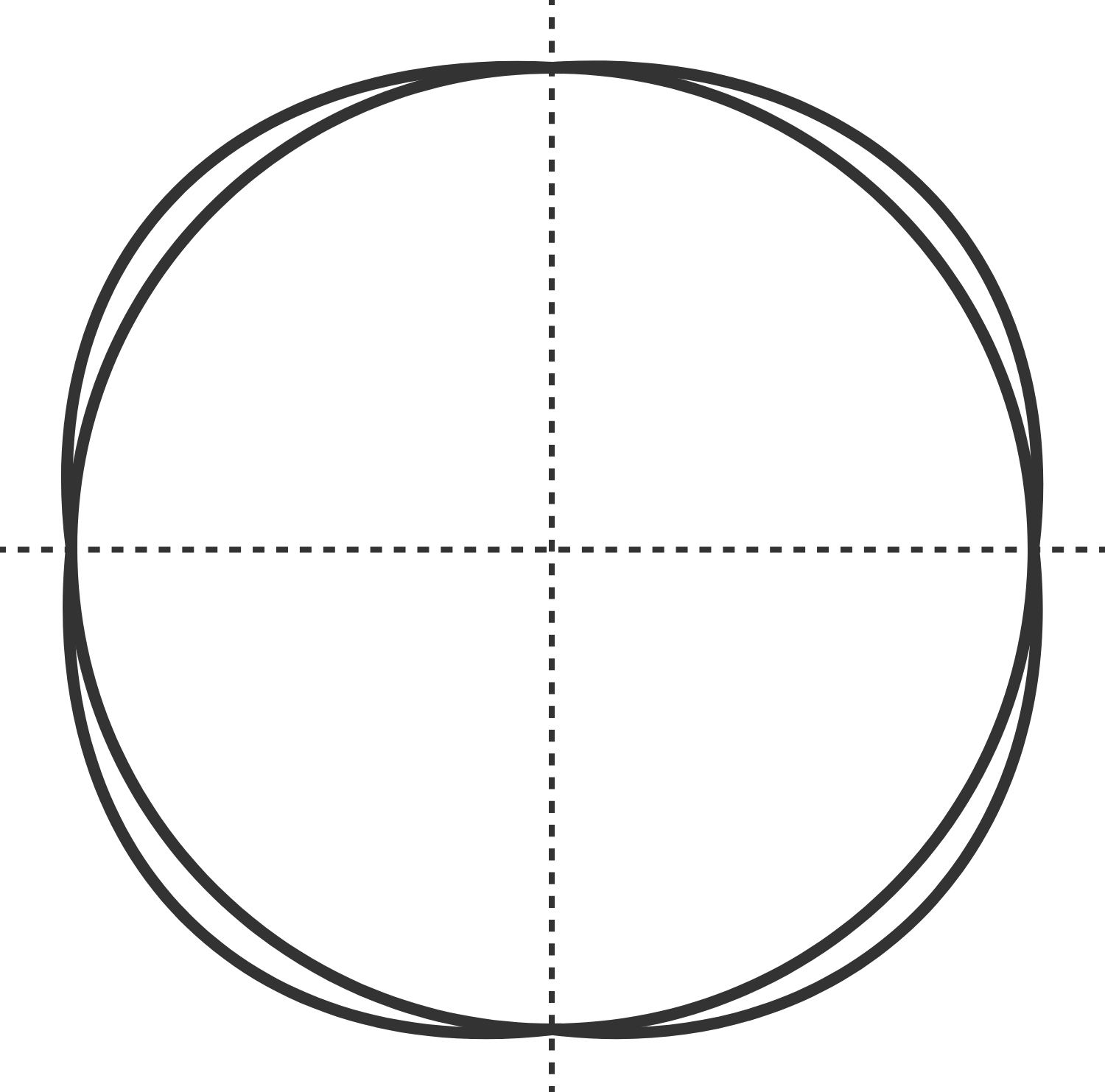# Bigger than a circle

Calculus Level 4The diagram above shows both a unit circle and a circle-like shape $S$--slightly larger than the unit circle--defined by the equation $\sqrt{1-|x|} + \sqrt{1-|y|} = 1.$ What is the percentage by which the area of $S$ is bigger than the area of the unit circle?